## Saturday, January 31, 2009

### What is a voltage divider? Pt3

Part 1
of this post showed a voltage divider made with two resistors connected end to end: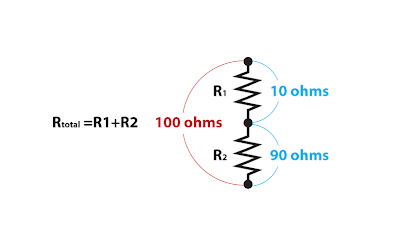It's a useful circuit, but it's action is fixed - determined be the value of the resistors chosen for the circuit. A potentiometer (commonly called a "pot") is a variable resistor and it can easily be wired to form a variable voltage divider.

A pot generally has three pins. The outer two pins connect to the opposites ends of the pot's resistive track. The resistance from one end of a pot to the other never changes. In this example it's 500 ohms.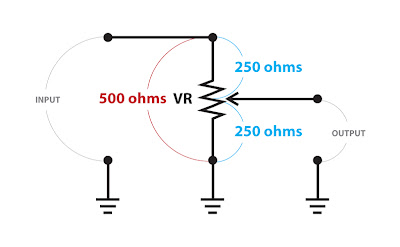The pot's center pin is connected to the wiper. The wiper moves along the resistive track as the pot is turned. Because the wiper simply splits total resistance into two parts, the sum of those two resistances is always equal to the total resistance from end to end. In this case 250 ohms + 250 ohms = 500 ohms.

To the circuit this looks like two 250 ohm resistors connected in series. Putting volts across the ends of the pot as we did in part 1 causes 1.5 volts to appear from each end of the pot to the wiper.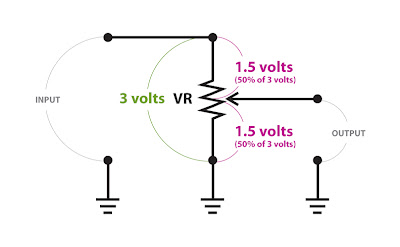So what happens when the pot is turned?

The resistance from end to end stays the same but the way that resistance is divided by the wiper changes: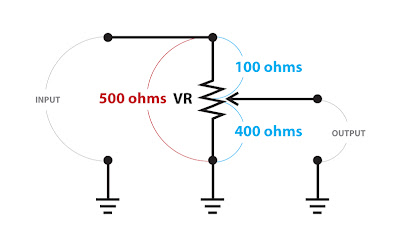Here the position of the wiper makes the pot function like a 100 ohm and a 400 ohm resistor connected in series. Following the rules from part 1 again, 3 volts in will produce 2.4 volts out.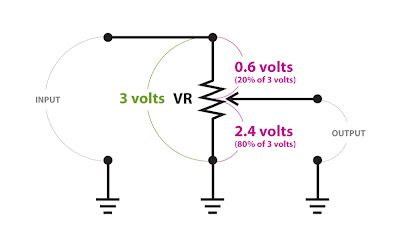If the pot is turned toward the other end of it's rotation and the resistances are reversed:Now 3 volts at the input yields only 0.6 volts at the output:This is just what is happening when you turn down the volume knob on your guitar or amplifier.

The Epiphone Valve Junior has both a fixed voltage divider for reducing the gain between stages and a variable voltage divider (the volume pot) wired in parallel. In the next post I'll take a look at that circuit as a real world example.